Gödel incompleteness theorem

A common name given to two theorems established by K. Gödel . Gödel's first incompleteness theorem states that in any consistent formal system containing a minimum of arithmetic (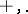, the symbols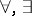, and the usual rules for handling them) a formally-undecidable proposition can be found, i.e. a closed formulasuch that neithernor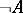can be deduced within the system. Gödel's second incompleteness theorem states that if certain natural completeness conditions are met, one can take this formulato be the formula which expresses the consistency of the system. These theorems indicated the failure of Hilbert's program on the foundations of mathematics, which expected a full formalization of all existing mathematics, or at least of a substantial part of it (Gödel's first incompleteness theorem proved that this is not possible), and attempted to justify the resulting formal system by a finite demonstration of its consistency (Gödel's second incompleteness theorem showed that even if all the tools of formalized arithmetic are considered to be finitary, this is still insufficient to prove the consistency of arithmetic).
One fixes a numbering (encoding) of the basic formal objects (formulas, finite sequences of formulas, etc.) by natural numbers so that the principal properties of these objects (to be an axiom or a logical derivation (cf. Derivation, logical) in accordance with the rules of the system, etc.) can be expressed by simple numbers. The calculation of the codes of results of combinatory transformations (e.g. substitution of a term for a variable in a formula) from the codes of the initial data are just as simple. It is possible, in this way, to write down an arithmetical formula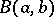of the form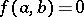, whereis a primitive recursive function, in order to express the following condition:is the code of the formula which is obtained from the formula with the codeby substitution of the natural numberfor the variable. Ifis the code of the formula, the formula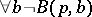denotes its own non-deducibility. It is thus formally undecidable. It follows that any consistent system with minimal arithmetical possibilities contains a true, but non-deducible statement of this type.
Gödel's second incompleteness theorem is obtained by formalizing the proof of Gödel's first incompleteness theorem. This proof involves a substantial use of the properties of the arithmetization of the syntax of the system under consideration, in that formulas expressing the following statements must be deducible: 1) the system is closed with respect to the rule of contraction of the argument (modus ponens); 2) the system is closed under substitution of terms for individual variables; and 3) the truth of a formula of a form, whereis a natural number andis a primitive recursive function, implies its deducibility. These conditions are met for natural arithmetization, but it is possible, without altering the algorithmic features of the arithmetization (all functions and predicates remain primitive recursive), to alter it so that the formula expressing the consistency of the system (with respect to the new arithmetization) becomes deducible. In the new arithmetization, condition 1) is violated.
Gödel's second incompleteness theorem gives a criterion for the comparison of formal systems: If it is possible to prove the consistency of a systemin a system, then the latter system cannot be interpreted in the former. It can thus be proved that formal mathematical analysis is not interpretable in arithmetic, the theory of types is not interpretable in analysis (cf. Types, theory of), and set theory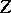is not interpretable in the theory of types.